# LBNF reference¶

## Introduction¶

This document defines the grammar formalism Labelled BNF (LBNF), which is used in the compiler construction tool BNF Converter. Given a grammar written in LBNF, the BNF Converter produces a complete compiler front end (up to, but excluding, type checking), i.e. a lexer, a parser, and an abstract syntax definition. Moreover, it produces a pretty-printer and a language specification in LaTeX, as well as a template file for the compiler back end. Since LBNF is purely declarative, these files can be generated in any programming language that supports appropriate compiler front-end tools. As of Version 2.0, code can be generated in Haskell, Java, C++, and C. This document describes the LBNF formalism independently of code generation, and is aimed to serve as a manual for grammar writers.

## A first example of LBNF grammar¶

As the first example of LBNF, consider a triple of rules defining addition expressions with “1”:

```EPlus. Exp ::= Exp "+" Num ;
ENum.  Exp ::= Num ;
NOne.  Num ::= "1" ;
```

Apart from the labels, EPlus, ENum, and NOne, the rules are ordinary BNF rules, with terminal symbols enclosed in double quotes and nonterminals written without quotes. The labels serve as constructors for syntax trees.

From an LBNF grammar, the BNF Converter extracts an abstract syntax and a concrete syntax. In Haskell, for instance, the abstract syntax is implemented as a system of datatype definitions

```data Exp = EPlus Exp Exp | ENum Num
data Num = NOne
```

For other languages—C, C++, and Java—an equivalent representation is given, following the methodology defined in Appel’s books series Modern compiler implementation in ML/Java/C . The concrete syntax is implemented by the lexer, parser and pretty-printer algorithms, which are defined in other generated program modules.

## LBNF in a nutshell¶

### Basic LBNF¶

Briefly, an LBNF grammar is a BNF grammar where every rule is given a label. The label is used for constructing a syntax tree whose subtrees are given by the nonterminals of the rule, in the same order.

More formally, an LBNF grammar consists of a collection of rules, which have the following form (expressed by a regular expression; Appendix gives a complete BNF definition of the notation): Ident ”.” Ident ”::=” (IdentString)* ”;” ; The first identifier is the rule label, followed by the value category. On the right-hand side of the production arrow (::=) is the list of production items. An item is either a quoted string (terminal) or a category symbol (non-terminal). A rule whose value category is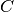is also called a production for.

Identifiers, that is, rule names and category symbols, can be chosen ad libitum, with the restrictions imposed by the target language. To satisfy Haskell, and C and Java as well, the following rule is imposed An identifier is a nonempty sequence of letters, starting with a capital letter.

Basic LBNF as defined above is clearly sufficient for defining any context-free language. However, it is not always convenient to define a programming language purely with BNF rules. Therefore, some additional features are added to LBNF: abstract syntax conventions, lexer rules, pragmas, and macros. These features are treated in the subsequent sections.

Section Abstract syntax conventions explains abstract syntax conventions. Creating an abstract syntax by adding a node type for every BNF rule may sometimes become too detailed, or cluttered with extra structures. To remedy this, we have identified the most common problem cases, and added to LBNF some extra conventions to handle them.

Section Lexer Definitions explains lexer rules. Some aspects of a language belong to its lexical structure rather than its grammar, and are more naturally described by regular expressions than by BNF rules. We have therefore added to LBNF two rule formats to define the lexical structure: tokens and comments.

Section LBNF Pragmas explains pragmas. Pragmas are rules instructing the BNFC grammar compiler to treat some rules of the grammar in certain special ways: to reduce the number of entrypoints or to treat some syntactic forms as internal only.

Section LBNF macros explains macros. Macros are syntactic sugar for potentially large groups of rules and help to write grammars concisely. This is both for the writer’s and the reader’s convenience; among other things, macros naturally force certain groups of rules to go together, which could otherwise be spread arbitrarily in the grammar.

Section Layout syntax explains layout syntax, which is a non-context-free feature present in some programming languages. LBNF has a set of rule formats for defining a limited form of layout syntax. It works as a preprocessor that translates layout syntax into explicit structure markers.

## Abstract syntax conventions¶

### Predefined basic types¶

The first convention are predefined basic types. Basic types, such as integer and character, can of course be defined in a labelled BNF, for example:

```Char_a. Char ::= "a" ;
Char_b. Char ::= "b" ;
```

This is, however, cumbersome and inefficient. Instead, we have decided to extend our formalism with predefined basic types, and represent their grammar as a part of lexical structure. These types are the following, as defined by LBNF regular expressions (see [reg] for the regular expression syntax):

• Integer of integers, defined digit+
• Double of floating point numbers, defined digit+ '.' digit+ ('e' '-'? digit+)?
• Char of characters (in single quotes), defined '\'' ((char - ["'\\"]) | ('\\' ["'\\nt"])) '\''
• String of strings (in double quotes), defined '"' ((char - ["\"\\"]) | ('\\' ["\"\\nt"]))* '"'
• Ident of identifiers, defined letter (letter | digit | '_' | '\'')*

In the abstract syntax, these types are represented as corresponding types of each language, except Ident, for which no such type exists. It is treated by a newtype in Haskell,:

```newtype Ident = Ident String
```

as String in Java, and as a typedef to char\* in C and C++.

As the names of the types suggest, the lexer produces high-precision variants, for integers and floats. Authors of applications can truncate these numbers later if they want to have low precision instead.

Predefined categories may not have explicit productions in the grammar, since this would violate their predefined meanings.

### Semantic dummies¶

Sometimes the concrete syntax of a language includes rules that make no semantic difference. An example is a BNF rule making the parser accept extra semicolons after statements:

```Stm ::= Stm ";" ;
```

As this rule is semantically dummy, we do not want to represent it by a constructors in the abstract syntax. Instead, we introduce the following convention:

A rule label can be an underscore _, which does not add anything to the syntax tree.

Thus we can write the following rule in LBNF:

```_ . Stm ::= Stm ";" ;
```

Underscores are of course only meaningful as replacements of one-argument constructors where the value type is the same as the argument type. Semantic dummies leave no trace in the pretty-printer. Thus, for instance, the pretty-printer “normalizes away” extra semicolons.

### Precedence levels¶

A common idiom in (ordinary) BNF is to use indexed variants of categories to express precedence levels:

```Exp3 ::= Integer ;
Exp2 ::= Exp2 "*" Exp3 ;
Exp  ::= Exp  "+" Exp2 ;
Exp  ::= Exp2 ;
Exp2 ::= Exp3 ;
Exp3 ::= "(" Exp ")" ;
```

The precedence level regulates the order of parsing, including associativity. Parentheses lift an expression of any level to the highest level.

A straightforward labelling of the above rules creates a grammar that does have the desired recognition behavior, as the abstract syntax is cluttered with type distinctions (between Exp, Exp2, and Exp3) and constructors (from the last three rules) with no semantic content. The BNF Converter solution is to distinguish among category symbols those that are just indexed variants of each other:

A category symbol can end with an integer index (i.e. a sequence of digits), and is then treated as a type synonym of the corresponding non-indexed symbol.

Thus Exp2 and Exp3 are indexed variants of Exp. The plain Exp is treated in the same way as Exp0.

Transitions between indexed variants are semantically dummy, and we do not want to represent them by constructors in the abstract syntax. To do this, we extend the use of underscores to indexed variants. The example grammar above can now be labelled as follows:

```EInt.   Exp3 ::= Integer ;
ETimes. Exp2 ::= Exp2 "*" Exp3 ;
EPlus.  Exp  ::= Exp  "+" Exp2 ;
_.      Exp  ::= Exp2 ;
_.      Exp2 ::= Exp3 ;
_.      Exp3 ::= "(" Exp ")" ;
```

In Haskell, for instance, the datatype of expressions becomes simply

```data Exp = EInt Integer | ETimes Exp Exp | EPlus Exp Exp
```

and the syntax tree for 2 * ( 3 + 1 ) is

```ETimes (EInt 2) (EPlus (EInt 3) (EInt 1))
```

Indexed categories can be used for other purposes than precedence, since the only thing we can formally check is the type skeleton (see the section The type-correctness of LBNF rules). The parser does not need to know that the indices mean precedence, but only that indexed variants have values of the same type. The pretty-printer, however, assumes that indexed categories are used for precedence, and may produce strange results if they are used in some other way.

Hint

See Section Coercions for a concise way of defining dummy coercions rules.

### Polymorphic lists¶

It is easy to define monomorphic list types in LBNF:

```NilDef.  ListDef ::= ;
ConsDef. ListDef ::= Def ";" ListDef ;
```

However, compiler writers in languages like Haskell may want to use predefined polymorphic lists, because of the language support for these constructs. LBNF permits the use of Haskell’s list constructors as labels, and list brackets in category names:

```[].  [Def] ::= ;
(:). [Def] ::= Def ";" [Def] ;
```

As the general rule, we have

[C], the category of lists of type C,

[] and (:), the Nil and Cons rule labels,

(:[]), the rule label for one-element lists.

The third rule label is used to place an at-least-one restriction, but also to permit special treatment of one-element lists in the concrete syntax.

In the LaTeX document (for stylistic reasons) and in the Happy file (for syntactic reasons), the category name [C] is replaced by ListX. In order for this not to cause clashes, ListX may not be at the same time used explicitly in the grammar.

The list category constructor can be iterated: [[X]], [[[X]]], etc behave in the expected way.

The list notation can also be seen as a variant of the Kleene star and plus, and hence as an ingredient from Extended BNF.

In other languages than Haskell, monomorphic variants of lists are generated automatically.

Hint

See Section Terminators and separators for concise ways of defining lists by just giving their terminators or separators.

### The type-correctness of LBNF rules¶

It is customary in parser generators to delegate the checking of certain errors to the target language. For instance, a Happy source file that Happy processes without complaints can still produce a Haskell file that is rejected by Haskell. In the same way, the BNF converter delegates some checking to the generated language (for instance, the parser conflict check). However, since it is always the easiest for the programmer to understand error messages related to the source, the BNF Converter performs some checks, which are mostly connected with the sanity of the abstract syntax.

The type checker uses a notion of the category skeleton of a rule, which is a pair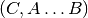whereis the unindexed left-hand-side non-terminal and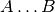is the sequence of unindexed right-hand-side non-terminals of the rule. In other words, the category skeleton of a rule expresses the abstract-syntax type of the semantic action associated to that rule.

We also need the notions of a regular category and a regular rule label. Briefly, regular labels and categories are the user-defined ones. More formally, a regular category is none of [C], Integer, Double, Char, String and Ident, or the types defined by token rules (Section The token rule). A regular rule label is none of _, , (:), and (:[]).

The type checking rules are now the following:

A rule labelled by _ must have a category skeleton of form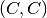.

A rule labelled by [] must have a category skeleton of form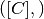.

A rule labelled by (:) must have a category skeleton of form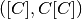.

A rule labelled by (:[]) must have a category skeleton of form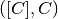.

Only regular categories may have productions with regular rule labels.

Every regular category occurring in the grammar must have at least one production with a regular rule label.

All rules with the same regular rule label must have the same category skeleton.

The second-last rule corresponds to the absence of empty data types in Haskell. The last rule could be strengthened so as to require that all regular rule labels be unique: this is needed to guarantee error-free pretty-printing. Violating this strengthened rule currently generates only a warning, not a type error.

## Lexer Definitions¶

### The token rule¶

The token rule enables the LBNF programmer to define new lexical types using a simple regular expression notation. For instance, the following rule defines the type of identifiers beginning with upper-case letters.

```token UIdent (upper (letter | digit | '_')*) ;
```

The type UIdent becomes usable as an LBNF nonterminal and as a type in the abstract syntax. Each token type is implemented by a newtype in Haskell, as a String in Java, and as a typedef to char* in C/C++.

The regular expression syntax of LBNF is specified in the Appendix. The abbreviations with strings in brackets need a word of explanation:

["abc7%"] denotes the union of the characters ‘ab‘ ‘c‘ ‘7‘ ‘%

{"abc7%"} denotes the sequence of the characters ‘a‘ ‘b‘ ‘c‘ ‘7‘ ‘%

The atomic expressions upper, lower, letter, and digit denote the character classes suggested by their names (letters are isolatin1). The expression char matches any character in the 8-bit ASCII range, and the “epsilon” expression eps matches the empty string.  Thus eps is equivalent to {""}, whereas the empty language is expressed by [""].

Note

The empty language is not available for the Java lexer tool JLex.

### The position token rule¶

(As of February 7, 2011, only available for Haskell). Any token rule can be modified by the word position, which has the effect that the datatype defined will carry position information. For instance,

```position token PIdent (letter (letter|digit|'_'|'\'')*) ;
```

creates in Haskell the datatype definition

```newtype PIdent = PIdent ((Int,Int),String)
```

where the pair of integers indicates the line and column of the first character of the token. The pretty-printer omits the position component.

### The comment rule¶

Comments are segments of source code that include free text and are not passed to the parser. The natural place to deal with them is in the lexer. The comment rule instructs the lexer generator to treat certain pieces of text as comments.

The comment rule takes one or two string arguments. The first string defines how a comment begins. The second, optional string marks the end of a comment; if it is not given then the comment is ended by a newline. For instance, the Java comment convention is defined as follows:

```comment "//" ;
comment "/*" "*/" ;
```

## LBNF Pragmas¶

### Internal pragmas¶

Sometimes we want to include in the abstract syntax structures that are not part of the concrete syntax, and hence not parsable. They can be, for instance, syntax trees that are produced by a type-annotating type checker. Even though they are not parsable, we may want to pretty-print them, for instance, in the type checker’s error messages. To define such an internal constructor, we use a pragma

```"internal" Rule ";"
```

where Rule is a normal LBNF rule. For instance,

```internal EVarT. Exp ::= "(" Ident ":" Type ")";
```

introduces a type-annotated variant of a variable expression.

### Entry point pragmas¶

The BNF Converter generates, by default, a parser for every category in the grammar. This is unnecessarily rich in most cases, and makes the parser larger than needed. If the size of the parser becomes critical, the entry points pragma enables the user to define which of the parsers are actually exported:

```entrypoints (Ident ",")* Ident ;
```

For instance, the following pragma defines Stm and Exp to be the only entry points:

```entrypoints Stm, Exp ;
```

## LBNF macros¶

### Terminators and separators¶

The terminator macro defines a pair of list rules by what token terminates each element in the list. For instance,

```terminator Stm ";" ;
```

tells that each statement (Stm) is terminated with a semicolon (;). It is a shorthand for the pair of rules

```[].  [Stm] ::= ;
(:). [Stm] ::= Stm ";" [Stm] ;
```

The qualifier nonempty in the macro makes one-element list to be the base case. Thus

```terminator nonempty Stm ";" ;
```

is shorthand for

```(:[]). [Stm] ::= Stm ";" ;
(:).   [Stm] ::= Stm ";" [Stm] ;
```

The terminator can be specified as empty "". No token is introduced then, but e.g.

```terminator Stm "" ;
```

is translated to

```[].  [Stm] ::= ;
(:). [Stm] ::= Stm [Stm] ;
```

The separator macro is similar to terminator, except that the separating token is not attached to the last element. Thus

```separator Stm ";" ;
```

means

```[].    [Stm] ::= ;
(:[]). [Stm] ::= Stm ;
(:).   [Stm] ::= Stm ";" [Stm] ;
```

whereas

```separator nonempty Stm ";" ;
```

means

```(:[]). [Stm] ::= Stm ;
(:).   [Stm] ::= Stm ";" [Stm] ;
```

Notice that, if the empty token "" is used, there is no difference between terminator and separator.

Problem. The grammar generated from a separator without nonempty will actually also accept a list terminating with a semicolon, whereas the pretty-printer “normalizes” it away. This might be considered a bug, but a set of rules forbidding the terminating semicolon would be much more complicated. The nonempty case is strict.

### Coercions¶

The coercions macro is a shorthand for a group of rules translating between precedence levels. For instance,

```coercions Exp 3 ;
```

is shorthand for

```_. Exp  ::= Exp1 ;
_. Exp1 ::= Exp2 ;
_. Exp2 ::= Exp3 ;
_. Exp3 ::= "(" Exp ")" ;
```

Because of the total coverage of these coercions, it does not matter if the integer indicating the highest level (here 3) is bigger than the highest level actually occurring, or if there are some other levels without productions in the grammar.

### Rules¶

The rules macro is a shorthand for a set of rules from which labels are generated automatically. For instance,

```rules Type ::= Type "[" Integer "]" | "float" | "double" | Type "*" ;
```

is shorthand for

```Type_0.      Type ::= Type "[" Integer "]" ;
Type_float.  Type ::= "float" ;
Type_double. Type ::= "double" ;
Type_3.      Type ::= Type "*" ;
```

The labels are created automatically. A label starts with the value category name. If the production has just one item, which is moreover possible as a part of an identifier, that item is used as a suffix. In other cases, an integer suffix is used. No global checks are performed when generating these labels. Any label name clashes that result from them are captured by BNFC type checking on the generated rules.

Notice that, using the rules macro, it is possible to define an LBNF grammar without giving any labels. To guarantee the uniqueness of labels, productions of the each category must be grouped together.

## Layout syntax¶

Layout syntax is a means of using indentation to group program elements. It is used in some languages, e.g. Haskell. Those who do not know what layout syntax is or who do not like it can skip this section.

The pragmas layout, layout stop, and layout toplevel define a layout syntax for a language. Before these pragmas were added, layout syntax was not definable in BNFC. The layout pragmas are only available for the files generated for Haskell-related tools; if Java, C, or C++ programmers want to handle layout, they can use the Haskell layout resolver as a preprocessor to their front end, before the lexer. In Haskell, the layout resolver appears, automatically, in its most natural place, which is between the lexer and the parser. The layout pragmas of BNFC are not powerful enough to handle the full layout rule of Haskell 98, but they suffice for the “regular” cases.

Here is an example, found in the the grammar of the logical framework Alfa.

```layout "of", "let", "where", "sig", "struct" ;
```

The first line says that "of", "let", "where", "sig", "struct" are layout words, i.e. start a layout list. A layout list is a list of expressions normally enclosed in curly brackets and separated by semicolons, as shown by the Alfa example

```ECase. Exp ::= "case" Exp "of" "{" [Branch] "}" ;

separator Branch ";" ;
```

When the layout resolver finds the token of in the code (i.e. in the sequence of its lexical tokens), it checks if the next token is an opening curly bracket. If it is, nothing special is done until a layout word is encountered again. The parser will expect the semicolons and the closing bracket to appear as usual.

But, if the tokenfollowing of is not an opening curly bracket, a bracket is inserted, and the start column ofis remembered as the position at which the elements of the layout list must begin. Semicolons are inserted at those positions. When a token is eventually encountered left of the position of(or an end-of-file), a closing bracket is inserted at that point.

Nested layout blocks are allowed, which means that the layout resolver maintains a stack of positions. Pushing a position on the stack corresponds to inserting a left bracket, and popping from the stack corresponds to inserting a right bracket.

Here is an example of an Alfa source file using layout:

```c :: Nat = case x of
True -> b
False -> case y of
False -> b
Neither -> d

d = case x of True -> case y of False -> g
x -> b
y -> h
```

Here is what it looks like after layout resolution:

```c :: Nat = case x of {
True -> b
;False -> case y of {
False -> b
};Neither -> d

};d = case x of {True -> case y of {False -> g
;x -> b
};y -> h} ;
```

There are two more layout-related pragmas. The layout stop pragma, as in

```layout stop "in" ;
```

tells the resolver that the layout list can be exited with some stop words, like in, which exits a let list. It is no error in the resolver to exit some other kind of layout list with in, but an error will show up in the parser.

The layout toplevel pragma tells that the whole source file is a layout list, even though no layout word indicates this. The position is the first column, and the resolver adds a semicolon after every paragraph whose first token is at this position. No curly brackets are added. The Alfa file above is an example of this, with two such semicolons added.

To make layout resolution a stand-alone program, e.g. to serve as a preprocessor, the programmer can modify the BNFC source file ResolveLayoutAlfa.hs as indicated in the file, and either compile it or run it in the Hugs interpreter by

```runhugs ResolveLayoutX.hs <X-source-file>
```

We may add the generation of ResolveLayoutX.hs to a later version of BNFC.

Bug. The generated layout resolver does not work correctly if a layout word is the first token on a line.

## Profiles¶

This section explains a feature which is not intended to be used in LBNF grammars written by hand, but in ones generated from the grammar formalism GF (Grammatical Framework). GF supports grammars of natural-languages and also higher-order abstract syntax which is sometimes used for formal languages to define their static semantics. The reader not familiar with these matters can skip this section.

The relation between abstract and concrete syntax in LBNF is the simplest possible one: the subtrees of an abstract syntax tree are in one-to-one correspondence with the nonterminals in the parsing grammar. The GF formalism generalizes this relation to one in which permutations, omissions, and duplications can occur in the transition from abstract and concrete syntax. The way back then requires a rearrangement of the subtrees, which involves unification in the case of omissions and duplications. It is also possible to conceive some concrete-syntax constituents as bound variables, as is the case in higher-order abstract syntax. The recipe for doing this postprocessing can be compactly expressed by a profile, which has a list of positions of each argument. For instance, the profiles in basic LBNF look as follows:

```While ([],)([],)([],). Stm ::= "while" "(" Exp ")" Stm Stm ;
```

That is, each abstract argument occurs exactly once in the concrete expression, and in the same order. The syntax trees produced have the form

```While Ext Stm Stm
```

The first components in each list of pairs are for variable bindings. An example is the variable declaration rule

```Decl ([],)([],). Stm ::= Typ Ident ";" Stm ;
```

which creates the abstract syntax

```Decl Typ (\Ident -> Stm)
```

An (artificial) example of duplication would be

```IsAlways ([],[0,1]). Sentence ::= "a" Noun "is" "always" "a" Noun  ;
```

which produces trees of the form

```IsAlways Noun
```

and would accept strings like a man is always a man, a bike is always a bike, but not a man is always a bike.

## An optimization: left-recursive lists¶

[leftrec]

The BNF representation of lists is right-recursive, following the list conctructor in Haskell and most other languages. Right-recursive lists, however, are an inefficient way of parsing lists in an LALR parser, because they can blow up the stack size. The smart programmer would implement a pair of rules such as

```[].    [Stm] ::= ;
(:).   [Stm] ::= Stm ";" [Stm] ;
```

not in the direct way, but under a left-recursive transformation, as if we wrote,

```[].         [Stm] ::= ;
(flip (:)). [Stm] ::= [Stm] Stm ";" ;
```

Then the smart programmer would also be careful to reverse the list when it is used as an argument of another rule construction.

The BNF Converter automatically performs the left-recursion transformation for pairs of rules of the form

```[].  [C] ::= ;
(:). [C] ::= C x [C] ;
```

where C is any category and x is any sequence of terminals (possibly empty). These rules can, of course, be generated from the terminator macro (Section [terminator]).

Notice. The transformation is currently not performed if the one-element list is the base case.

## Appendix: LBNF Specification¶

This document was automatically generated by the BNF-Converter. It was generated together with the lexer, the parser, and the abstract syntax module, which guarantees that the document matches with the implementation of the language (provided no hand-hacking has taken place).

## The lexical structure of BNF¶

### Identifiers¶

Identifiers are unquoted strings beginning with a letter, followed by any combination of letters, digits, and the characters _ ’, reserved words excluded.

### Literals¶

String literals  have the form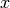, whereis any sequence of any characters except  unless preceded by \.

Integer literals  are nonempty sequences of digits.

Character literals  have the form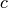, whereis any single character.

### Reserved words and symbols¶

The set of reserved words is the set of terminals appearing in the grammar. Those reserved words that consist of non-letter characters are called symbols, and they are treated in a different way from those that are similar to identifiers. The lexer follows rules familiar from languages like Haskell, C, and Java, including longest match and spacing conventions.

The reserved words used in BNF are the following:

```char        coercions   comment
digit       entrypoints eps
internal    layout      letter
lower       nonempty    position
rules       separator   stop
terminator  token       toplevel
upper
```

The symbols used in BNF are the following:

```; . ::=
[ ] _
( : )
, | -
* + ?
{ }
```

Multiple-line comments are enclosed with and .

## The syntactic structure of BNF¶

Non-terminals are enclosed between < and >. The symbols ::= (production), ::= (union) and ε (empty rule) belong to the BNF notation. All other symbols are terminals.

```<Grammar> ::= <ListDef>

<ListDef> ::= ε
|   <Def> ; <ListDef>
<ListItem> ::= ε
|   <Item> <ListItem>
<Def> ::= <Label> . <Cat> ::= <ListItem>
|   comment <String>
| comment <String> <String>
| internal <Label> . <Cat> ::= <ListItem>
| token <Ident> <Reg>
| position token <Ident> <Reg>
| entrypoints <ListIdent>
| separator <MinimumSize> <Cat> <String>
| terminator <MinimumSize> <Cat> <String>
| coercions <Ident> <Integer>
| rules <Ident> ::= <ListRHS>
| layout <ListString>
| layout stop <ListString>
| layout toplevel
<Item> ::= <String>
| <Cat>
<Cat> ::= [ <Cat> ]
| <Ident>
<Label> ::= <LabelId>
| <LabelId> <ListProfItem>
| <LabelId> <LabelId> <ListProfItem>
<LabelId> ::= <Ident>
| -
| [ ]
| ( : )
| ( : [ ] )
<ProfItem> ::= ( [ <ListIntList> ] , [ <ListInteger> ] )
<IntList> ::= [ <ListInteger> ]

<ListInteger> ::= ε
| <Integer>
| <Integer> , <ListInteger>
<ListIntList> ::= ε
| <IntList>
| <IntList> , <ListIntList>
<ListProfItem> ::= <ProfItem>
| <ProfItem> <ListProfItem>
<ListString> ::= <String>
| <String> , <ListString>
<ListRHS> ::= <RHS>
| <RHS> | <ListRHS>
<RHS> ::= <ListItem>
<MinimumSize> ::= nonempty
| ε
<Reg2> ::= <Reg2> <Reg3>
| <Reg3>

<Reg1> ::= <Reg1> | <Reg2>
| <Reg2> − <Reg2>
| <Reg2>
<Reg3> ::= <Reg3> *
| <Reg3> +
| <Reg3> ?
| eps
| <Char>
| [ <String> ]
| { <String> }
| digit
| letter
| upper
| lower
| char
| ( <Reg> )
<Reg> ::= <Reg1>
<ListIdent> ::= <Ident>
| <Ident> , <ListIdent>
```
  Cambridge University Press, 1998.
  If we want to describe full Java or Haskell, we must extend the character set to Unicode. This is currently not supported by all lexer tools, however.